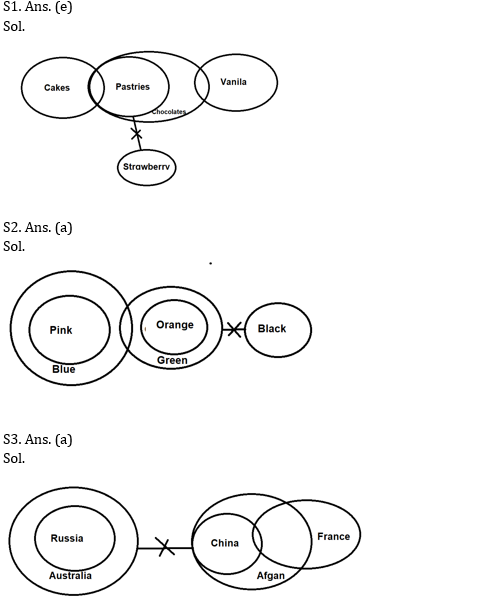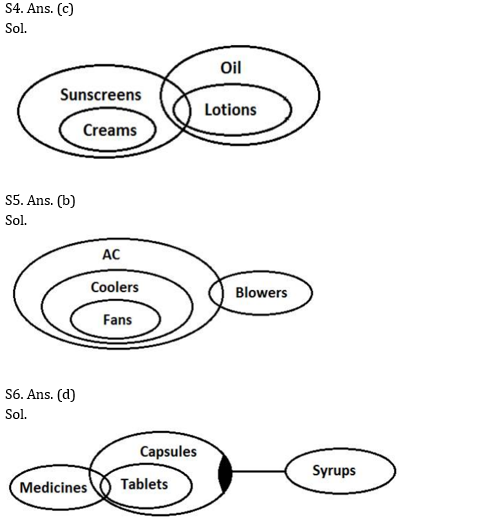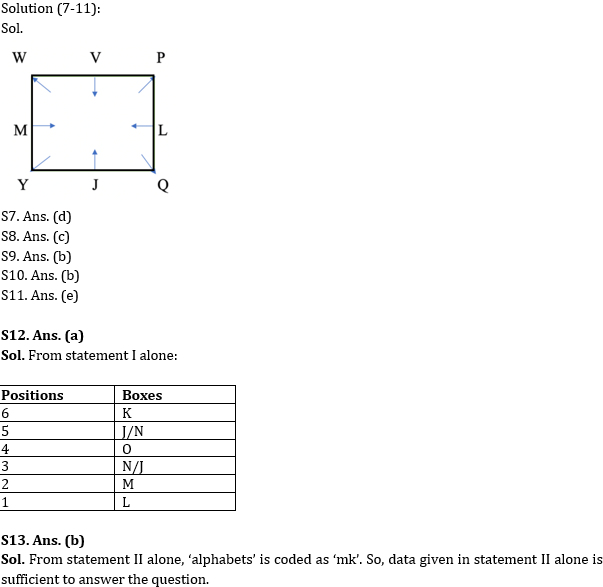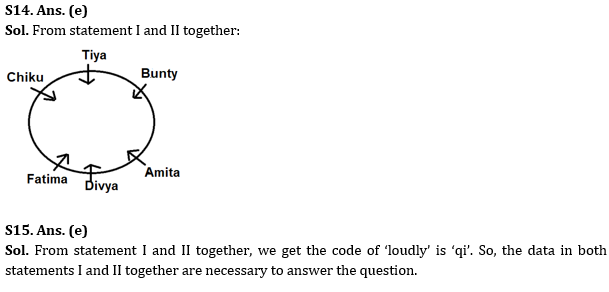Latest Banking jobs   »

# Reasoning Ability Quiz For RBI Grade B/ ECGC PO/ SIDBI Grade A Prelims 2022- 27th May

Directions (1-6): In each question, some statements are given followed by some conclusions. You have to take the given conclusion to be true even if they seem to be at variance with commonly known facts. Read all the conclusions and decide which of the given conclusions follow the given statements.

Q1. Statements: Some Cakes are Pastries. All Pastries are Chocolate. Some Chocolate is Vanila. No Stawberry is Pastries.
Conclusions:
I. All Vanila being Strawberry is a possibility.
II. All Strawberry being Cakes is a possibility.
(a) Only I follows
(b) Only II follows
(c) Either I or II follows
(d) Neither I nor II follow
(e) Both I and II follow

Q2. Statements: All Pinks is Blues. Some Greens are Blues. All Oranges are Greens. No Black is Green.
Conclusions:
I. No Black is Orange
II. Some Oranges are Blues
(a) Only I follows
(b) Only II follows
(c) Either I or II follows
(d) Neither I nor II follow
(e) Both I and II follow

Q3. Statements: All Russia are Australia. No Australia is China. All China are Afghans. Some China are France.
Conclusions:
I. All Australia being Afghans is a possibility.
II. Some France is Russia.
(a) Only I follows
(b) Only II follows
(c) Either I or II follows
(d) Neither I nor II follow
(e) Both I and II follow

Q4. Statements: All Creams are Sunscreens. Only a few Sunscreens are Lotions. All Lotions are Oil.
Conclusions:
I. Some Creams are Lotions
II. No Creams are Lotions
(a) Only I follows
(b) Only II follows
(c) Either I or II follows
(d) Neither I nor II follow
(e) Both I and II follow

Q5. Statements: All Fans are Coolers. All Coolers are AC. Only a few AC are Blowers.
Conclusions:
I. Some Blowers are Coolers
II. Some AC are Fans
(a) Only I follows
(b) Only II follows
(c) Either I or II follows
(d) Neither I nor II follow
(e) Both I and II follow

Q6. Statements: Only a few Medicines are Tablets. All Tablets are Capsules. Some Capsules are not Syrups.
Conclusions:
I. Some Tablets are not Syrups.
II. Some Capsules are not Medicines.
(a) Only I follows
(b) Only II follows
(c) Either I or II follows
(d) Neither I nor II follow
(e) Both I and II follow

Directions (7-11): Study the following information carefully and answer the questions accordingly.
Eight people sit around a square table. Four of them sit at the corners facing outside and the remaining four sit at the sides of the table facing inside. V sits opposite J who does not face outside. P is a neighbor of V and sits three places away from M. Q sits third to the right of M. Y sits second to the left of W. Y is not a neighbor of L.

Q7. Who among the following are the immediate neighbors?
(a) Y, Q
(b) M, L
(c) V, Q
(d) M, Y
(e) None of these

Q8. Who among the following sits second to the right of W?
(a) Y
(b) L
(c) P
(d) Q
(e) None of these

Q9. The one who sits opposite to J sits……………?
(a) Near M
(b) Immediate left of P
(c) Third to the right of L
(d) Immediate left of Q
(e) None of these

Q10. What is the sum of place values of the neighbors of Q?
(a) 26
(b) 22
(c) 36
(d) 43
(e) All are incorrect

Q11. Four among the following five are same in a certain manner and so form a group. Which is the one that does not belong to the group?
(a) W, Q
(b) Y, P
(c) M, L
(d) V, J
(e) Q, Y

Directions (12-15): The following question is followed by two statements I, and II. You have to decide, if these statements are sufficient to answer the asked questions and mark the correct option.

Q12. Six boxes, M, N, O, J, K and L are placed one above another, where the bottommost position is 1 and the position above it is 2 and so on. Which box is placed at the bottommost position?

Statements:
I. O is placed 2nd above M which is placed just above of L. One box is placed between N and J. J is placed below K.
II. Two boxes are placed between N and M. One box is placed between O and M.
(a) The data in statement I alone are sufficient to answer the question while the data in statement II alone are not sufficient to answer the question
(b) The data in statement II alone are sufficient to answer the question while the data in statement I alone are not sufficient to answer the question
(c) The data either in statement I alone or in statement II alone are sufficient to answer the question
(d) The data given in both statements I and II together are not sufficient to answer the question
(e) The data in both statements I and II together are necessary to answer the question

Q13. In the given code language, how is the word ‘alphabets’ coded?

Statements:
I. ‘Dividents followed by Assets’ is coded as ‘zx, ar, cp, lt’ and ‘coded alphabets and digits’ is coded as ‘mk, kr, dn, st’.
II. ‘Alphabets and Dividents numbers’ is coded as ‘lt, mk, ar, st’ and ‘digits with alphabets coded’ is coded as ‘dn, zx, mk, kr’
(a) The data in statement I alone are sufficient to answer the question, while the data in statement II alone are not sufficient to answer the question
(b) The data in statement II alone are sufficient to answer the question, while the data in statement I alone are not sufficient to answer the question
(c) The data either in statement I alone or in statement II alone are sufficient to answer the question
(d) The data given in both statements I and II together are not sufficient to answer the question
(e) The data in both statements I and II together are necessary to answer the question

Q14. Six girls namely, Amita, Bunty, Chiku, Divya, Tiya and Fatima sit around a circular table facing the centre. Who is second to the left of Bunty?

Statements:
I. Diya sits opposite to Tiya and is the immediate neighbor of Fatima and Amita. Chiku is third to the left of Amita.
II. Tiya is immediate neighbor of Bunty and Chiku. Fatima is second to the left of Amita.
(a) The data in statement I alone are sufficient to answer the question, while the data in statement II alone are not sufficient to answer the question
(b) The data in statement II alone are sufficient to answer the question, while the data in statement I alone are not sufficient to answer the question
(c) The data either in statement I alone or in statement II alone are sufficient to answer the question
(d) The data given in both statements I and II together are not sufficient to answer the question
(e) The data in both statements I and II together are necessary to answer the question

Q15. In the given coded language, how is the word ‘loudly’ coded?

Statements:
I. In a certain language, ‘they are fool’ is coded as ‘di ci ni’ and ‘fool loudly clearly’ is coded as ‘ni pi qi’.
II. In a certain language, ‘loudly part difference’ is coded as ‘qi ei fi’ and ‘precious difference slice’ is coded as ‘fi gi mi’
(a) The data in statement I alone are sufficient to answer the question, while the data in statement II alone are not sufficient to answer the question
(b) The data in statement II alone are sufficient to answer the question, while the data in statement I alone are not sufficient to answer the question
(c) The data either in statement I alone or in statement II alone are sufficient to answer the question
(d) The data given in both statements I and II together are not sufficient to answer the question
(e) The data in both statements I and II together are necessary to answer the question

Solutions#### Congratulations!